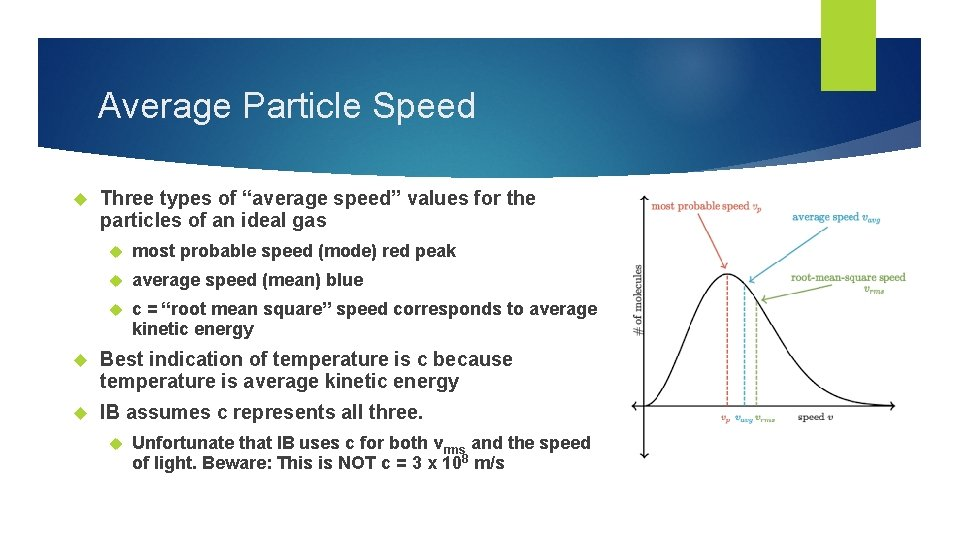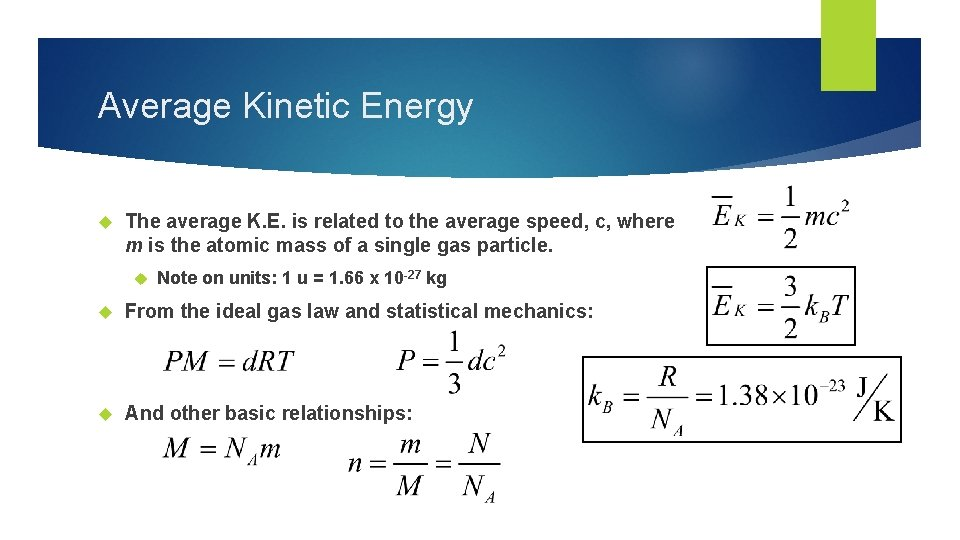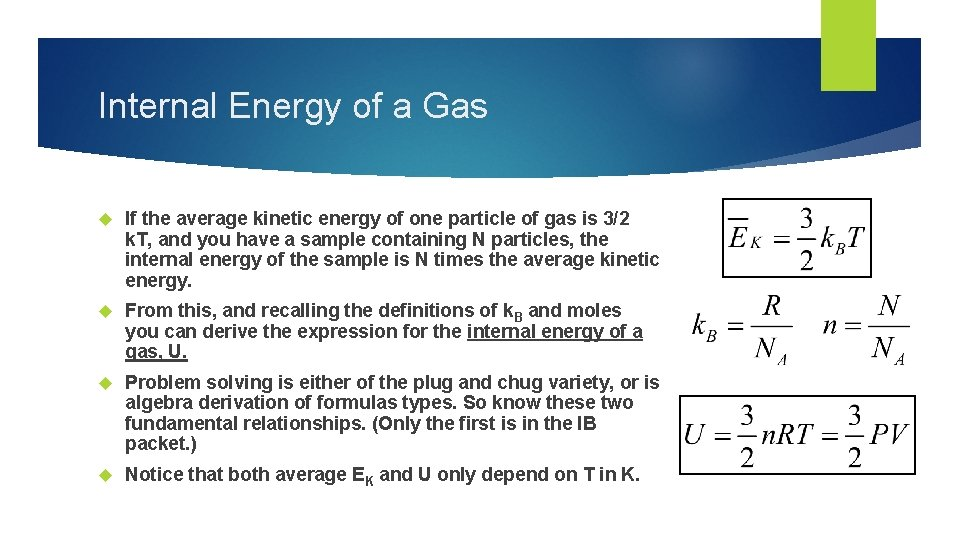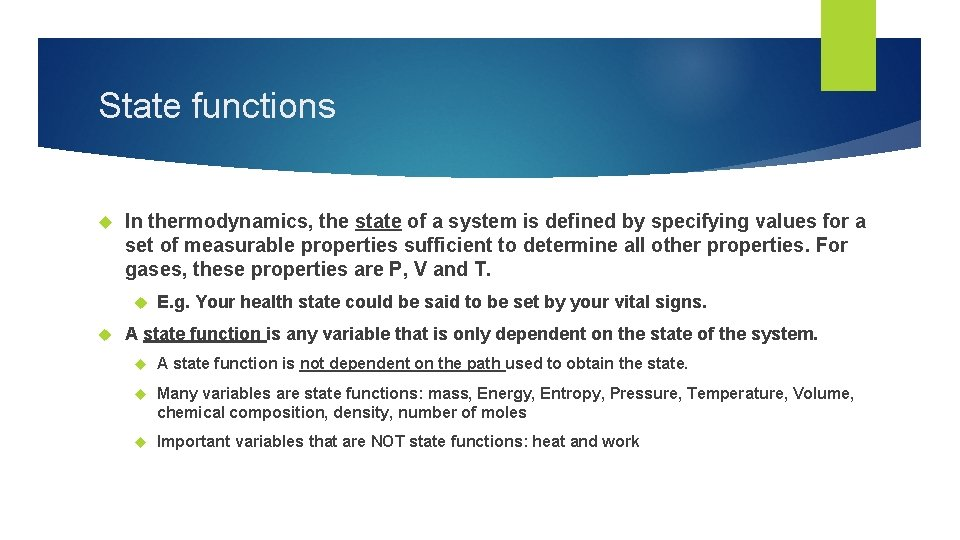# Physics 2 Feb 16 2017 P 3 Challenge

• Slides: 8Physics 2 – Feb 16, 2017 P 3 Challenge – What is the pressure of a canister of nitrogen gas (M=28 g/mole) if a 150 g sample has a volume of 68. 0 dm 3 at a temperature of 22. 0 C? Get out # 17 -29 for a HMK check Today’s Objective: B. 2. 1 Thermodynamics: Boltzmann Assignment: Ch 3. 2, p 140, #17 -29 Agenda Homework Review Boltzmann Distribution Average K. E. Internal Energy State functions Types of ProcessesBoltzmann Distribution Boltzmann distribution is a result of statistical mechanics that describes how the random particle speeds of an ideal gas are distributed. Note: Statistical mechanics is the application of statistics to the gas particle behavior. Unsymmetrical distribution. Always a finite probability at any high speed, but 0 at 0 speed. The ranking of temperature for the three graphs are Blue<Red<Green. Notice the peak location increases and lowers and the speeds spread out.Average Particle Speed Three types of “average speed” values for the particles of an ideal gas most probable speed (mode) red peak average speed (mean) blue c = “root mean square” speed corresponds to average kinetic energy Best indication of temperature is c because temperature is average kinetic energy IB assumes c represents all three. Unfortunate that IB uses c for both vrms and the speed of light. Beware: This is NOT c = 3 x 108 m/sAverage Kinetic Energy The average K. E. is related to the average speed, c, where m is the atomic mass of a single gas particle. Note on units: 1 u = 1. 66 x 10 -27 kg From the ideal gas law and statistical mechanics: And other basic relationships:Internal Energy of a Gas If the average kinetic energy of one particle of gas is 3/2 k. T, and you have a sample containing N particles, the internal energy of the sample is N times the average kinetic energy. From this, and recalling the definitions of k. B and moles you can derive the expression for the internal energy of a gas, U. Problem solving is either of the plug and chug variety, or is algebra derivation of formulas types. So know these two fundamental relationships. (Only the first is in the IB packet. ) Notice that both average EK and U only depend on T in K.State functions In thermodynamics, the state of a system is defined by specifying values for a set of measurable properties sufficient to determine all other properties. For gases, these properties are P, V and T. E. g. Your health state could be said to be set by your vital signs. A state function is any variable that is only dependent on the state of the system. A state function is not dependent on the path used to obtain the state. Many variables are state functions: mass, Energy, Entropy, Pressure, Temperature, Volume, chemical composition, density, number of moles Important variables that are NOT state functions: heat and workTypes of Processes A thermodynamic process is one that takes a system from one state to another. There are several types of processes dependent on the conditions for the change. (We’ve already seen most with the simple gas laws. ) Isothermal process – Temperature is constant e. g. Boyle’s law Isobaric process – Pressure is constant e. g. Charles’ law Isovolumetric process – Volume is constant Adiabatic process – No heat transfer e. g. Gay-Lussac’s LawExit Slip - Assignment Exit Slip- Ex: What is the internal energy of 3. 5 moles of oxygen gas at 298 K? What’s Due on Feb 16? (Pending assignments to complete. ) Ch 3. 2, p 141, 30 -32 DOWNLOAD Engineering Physics Text B!!!! Read B. 2. 1 -B. 2. 4, p 19 -23 What’s Next? (How to prepare for the next day) Read B p-32 about Thermodynamics